Chapter 19

The simplest helium atom consists of two protons and two electrons.
The helium atom’s electric charge and magnetic charge density distribution is: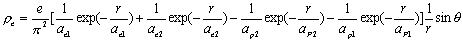(19.1)

Helium’s magnetic charge density distribution is:(19.2)

The helium atom’s electric charge distribution equation is: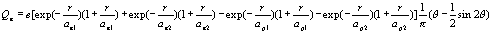(19.3)

Helium’s magnetic charge distribution equation is:(19.4)

Helium’s electric field equation is: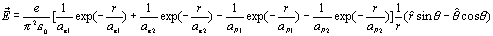(19.5)

The helium atom’s magnetic field equation is:(19.6)

Thus:(19.7)

As we know from (6.3), thus: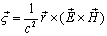(19.8)

Thus: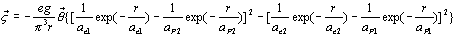(19.9)

For the cylindrical coordinates (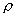,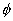, z), which has the following relationship with the spherical coordinate (r,,):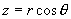We can separate the angular momentum density into ‘z’ component and ‘’ component,

The ‘z’ component of angular momentum density is: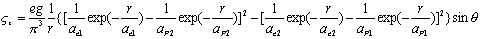(19.10)

The’ component of angular momentum density is: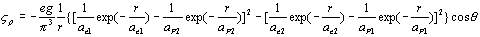(19.11)

As we know, the volume element is: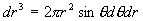For the ‘component of angular momentum density, because: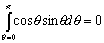Thus, we can get the ‘component of angular momentum: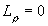(19.12)

For the ‘z’ component of electron angular momentum, we have: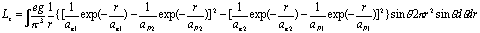(19.13)

Thus: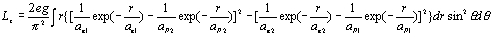(19.14)

Thus: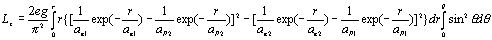(19.15)

Thus: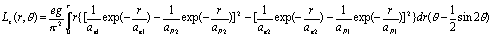(19.16)

When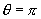, thus the z component of angular momentum is: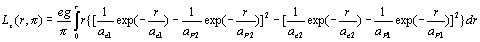(19.17)

When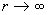, thus:(19.18)

In which(19.19)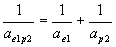(19.20)

As we know, eg=h

Thus: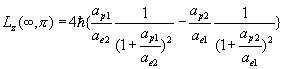(19.21)

As we know:(19.22)

And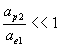(19.23)

Thus: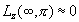(19.24)

The helium atom’s spin (angular momentum) is almost zero.